# Combine / add term

Combine like terms 4c+c-7c

Result

x = (Correct answer is: -2c)#### Solution:Leave us a comment of example and its solution (i.e. if it is still somewhat unclear...):Be the first to comment!## Next similar examples: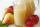Mam used 9 red, 9 green apples and 3 pears to prepare juice. How many pieces of fruit used by mom to prepare this juice?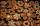John decorated 29 gingerbread and Annie 8 more. How many gingerbread decorated Annie?
3. Crocuses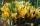The garden grow daffodils, crocuses and roses. 1400 daffodils, crocuses is 462 more and roses is 156 more than crocuses. How many are all the flowers in the garden?
4. Evaluate expression 2Evaluate expression with negatives: (-3)+4+(-8)+(-6)+4+(-1)
5. Adding mixed numerals3 3/4 + 2 3/5 + 5 1/2 Show your solution.
6. Trees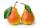In the orchard, 38 apple trees and 20 pear trees grew. How many trees there grow?
7. Roman numerals +Add up the number writtens in Roman numerals. Write the results as a decimal number.
8. Winnie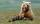Winnie Hugo had the right paw 10 punches and on 15 left more. How many punches has on left paw?
9. NoontimeIn the morning temperature was 110°F. By noontime it has gone up by 15°F. What was the noon temperature. (Give your answer in °F)
10. Sum of fractionsWhat is the sum of 2/3+3/5?
11. Roman numerals 2+Add up the number writtens in Roman numerals. Write the results as a roman numbers.
12. AverageThe arithmetic mean of the two numbers is 71.7. One number is 5. Calculate the second number.
13. BicycleThe bicycle pedal gear has 36 teeth, the rear gear wheel has 10 teeth. How many times turns rear wheel, when pedal wheel turns 120x?
14. ClassIn 7.C clss are 10 girls and 20 boys. Yesterday was missing 20% of girls and 50% boys. What percentage of students missing?
15. Obtuse angleWhich obtuse angle is creating clocks at 17:00?
16. IronIron ore contains 57% iron. How much ore is needed to produce 20 tons of iron?
17. Seeds 2How many seeds germinated from 1000 pcs, when 23% no emergence?